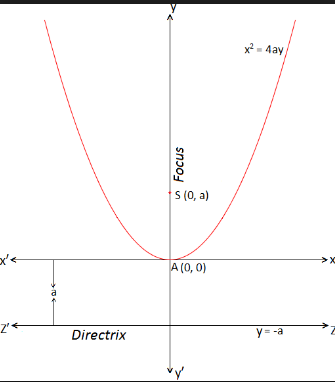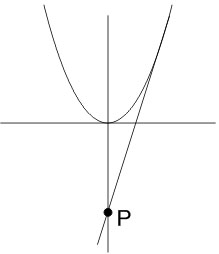#### If the common tangents to the parabola, x2=4y and the circle, x2+y2=4 intersect at the point P, then the distance of P from the origin, is : Option 1)Option 2)Option 3)Option 4)As we learnt in

Standard equation of parabola -- whereinCondition of tangency -- wherein

Ifis a tangent to the circleTangent toisAlsoIf we put D=0Option 1)This option is incorrect

Option 2)This option is incorrect

Option 3)This option is correct

Option 4)This option is incorrect

#### Vakul